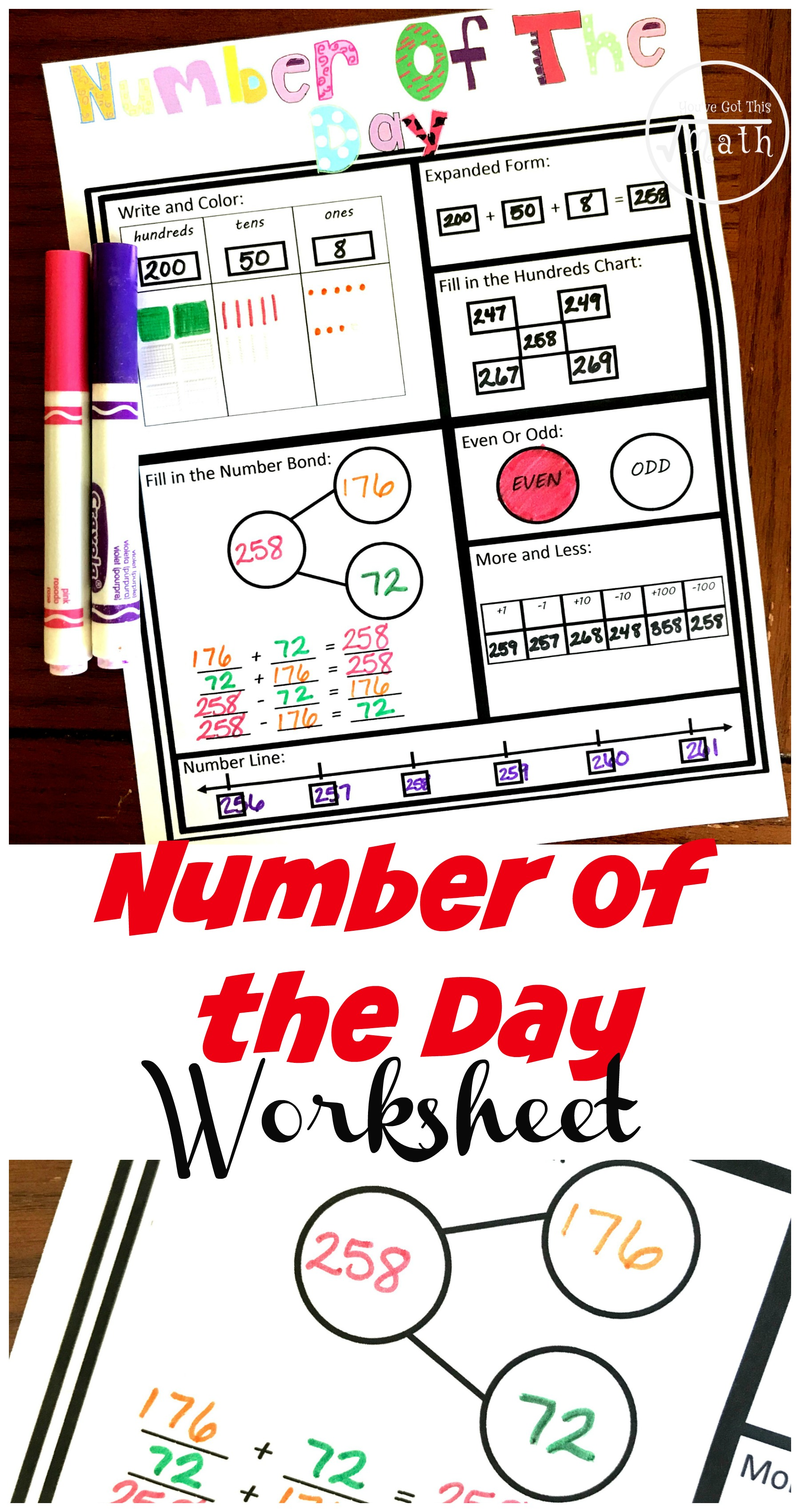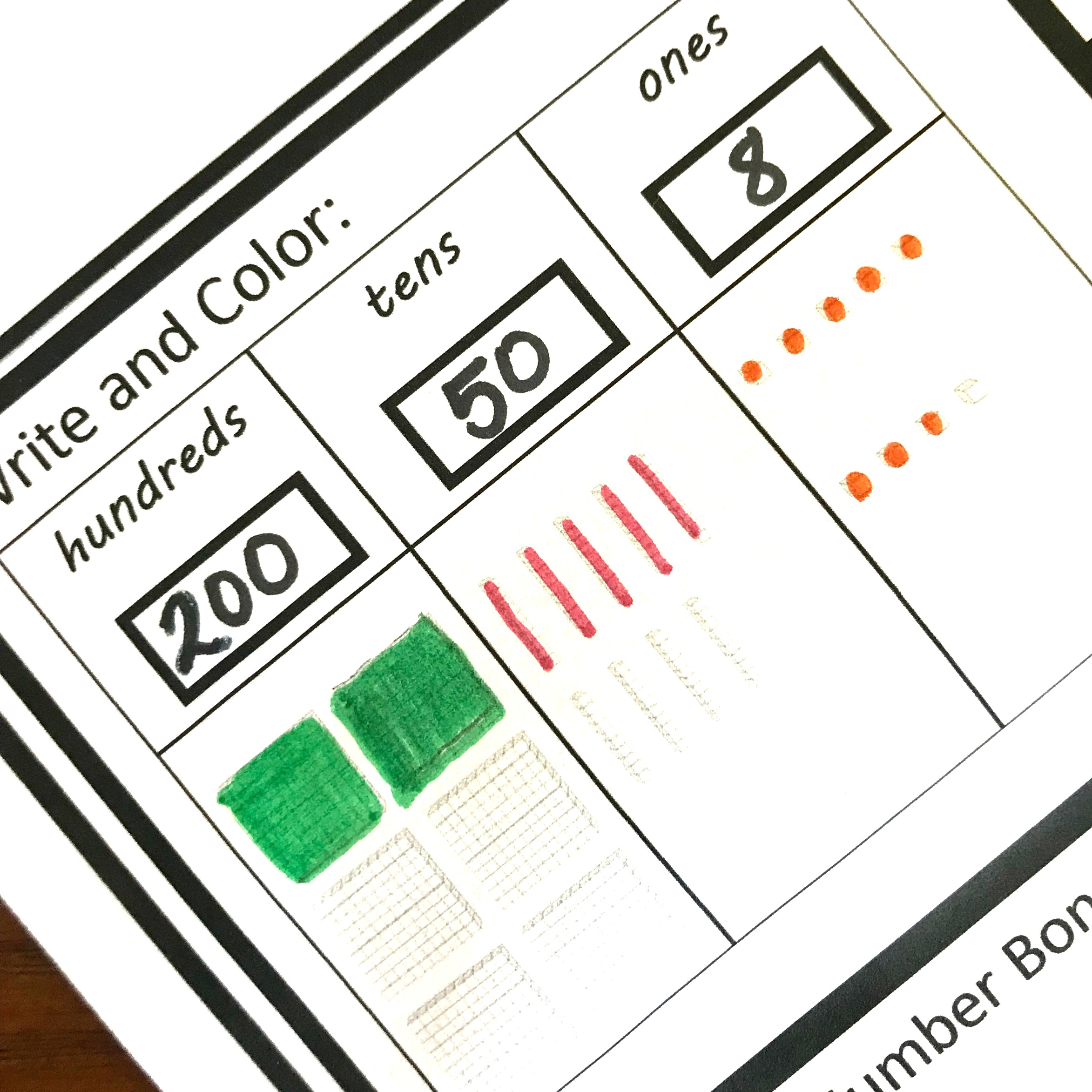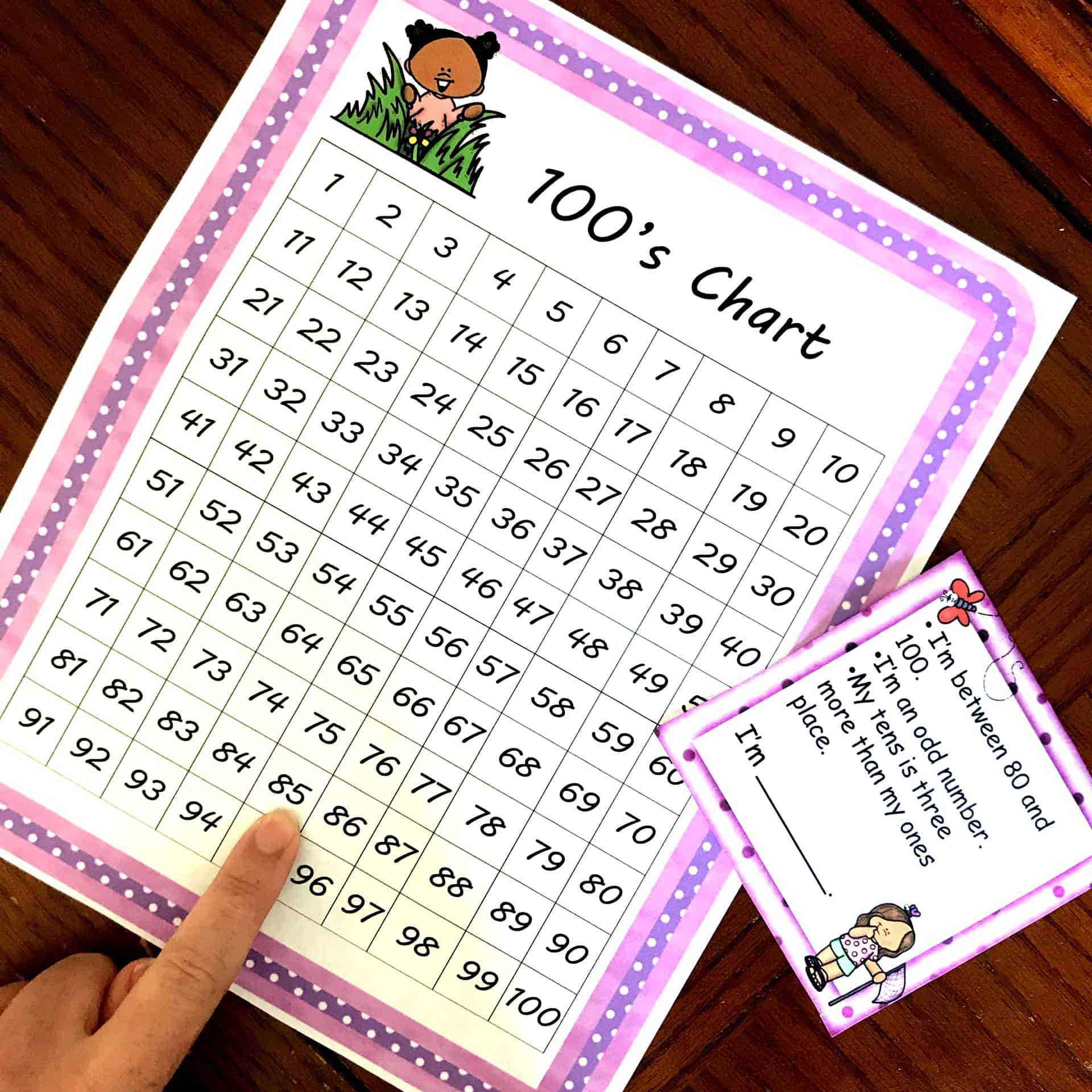Home » Math » Number of the Day

Number of the Day

This number of the day worksheet has children working on a hundreds chart, coloring in base ten blocks, filling in number lines, writing a number in expanded form and more.You can download this free printable by clicking on the blue button at the very END of this post.

Place value! It an essential part of every math curriculum. For children to be really successful in math they MUST understand this….or they will just be going through the motions. Motions that are not understood, and will be forgotten as they move on.

There are many ways that you can teach place value, and doing small place value activities daily is important. So today I’m sharing with you a number of the day worksheet that will help you develop place value skills each and every day.

You can also get a  Fractions of the Day, or a Decimals of the Day

Number of the Day Worksheet: Base Ten BlocksThe first part of this sheet is to color in the base ten blocks that represent the number of the day and to also write how many hundreds, tens, and ones there are.

In this example, the student was given the number 258. As you can see they wrote that there were two hundreds, and then colored in two flats.

Next, they looked in the tens place and realized that there were 5 tens or 50. The student was then able to color in five longs.

Finally, they looked at the ones place and saw that there were eight ones. They wrote 8 in the ones place and then colored in 8 cubes.

Expanded Form

Once this is done, they jump right to expanded form, and this is easy since we just figured out how many hundreds, tens, and ones were in the number 258.

All they need to do is put it together. 200 + 50 + 8 equals 258.

Hundreds ChartRight underneath expanded form, we move to a funny looking grid. This is a hundred’s chart puzzle, and if they haven’t done these before it may be a great idea to practice with our Hundreds Puzzle game.

The student can put the number 258 anywhere in the grid, and then figure out what the rest of the numbers would be if this was a hundred chart.

In this example, the child put the 258 right in the middle. They recognized that going up one space would put them at 248, and the number to the left would be 247 and the number to the right would be 249. Now the student used the same strategy going down. They added 10, and the subtracted and add one from 268 to get the next two answers.

There is so much math happening here!!!

Number BondsMoving on to our next section, we hit number bonds. This is a wonderful way to work on fact families in a very visual way. The children figure out two numbers that equal 258, and then they fill out the number bonds and the fact families that correspond to those numbers.

If you are working with young children and want to introduce number bonds, these monster task cards are a great place to start.

Even and OddThe next one is an easy one. Is the number even or odd? Once they figure that out, they just color in the circle.

More And Less

Understanding one more and one less, and ten more or less is an important skill.  This reinforces the place value of each digit…..and this next box tackles this concept.

The children look at the number 258 and then do each of the following.

First, we do 258 + 1 = 259

Next comes 258 – 1 = 257

We move on to 258 + 10 = 268

And we end with 258 – 10 = 248

Get more adding and subtracting by 10 activities here.

Number Line

The last step of this number of the day worksheet is to put numbers on the number line. You could allow children to write the Number of the Day in the first box and move on from there….but you can definitely make it harder.

Asking them to put the number of the day in the middle or at the end of the number line will add a challenge. Now they must be able to count backward as well as count forward! A great place value lesson!Working through a number of the day daily will help children build number sense and develop a deeper understanding of place value.

You’ve Got This,

Rachel

You may also like these hundreds chart riddles:This is a subscriber freebie. If you are already a subscriber, check the most recent email for the latest password. If you aren’t a subscriber, sign up below and the password will be provided in the first email you receive. You will also get \$2 off to use the You’ve Got This Store.

You’ll find it under the Building Number Sense Section!